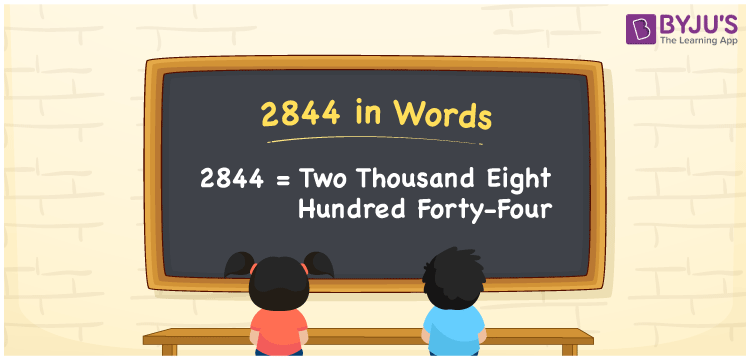# 2844 in Words

The numeral 2844 in words “two thousand eight hundred forty-four”. The number 2844 is a cardinal number. For example, the cost of 15 books is Rs. 2844. To convert the number 2844 into words, we usually prefer the place value system. Now, let us have a look at the method of writing the number 2844 in words in this article.

 2844 in Words: Two Thousand Eight Hundred Forty-four. Two Thousand Eight Hundred Forty-four in Numerical Form: 2844.

## 2844 in English Words## How to Write 2844 in Words?

The following table presents the place values of the number 2844.

 Thousands Hundreds Tens Ones 2 8 4 4

The expanded form of 2844 is as follows:

= 2 × Thousand + 8 × Hundred + 4 × Ten + 4 × One

= 2 × 1000 + 8 × 100 + 4 × 10 + 4 × 1

= 2000 + 800 + 40 + 4

= 2844

= Two thousand eight hundred forty-four

Hence, 2844 in words is two thousand eight hundred forty-four.

2844 in words – Two thousand eight hundred forty-four

Is 2844 an odd number? – No

Is 2844 an even number? – Yes

Is 2844 a perfect square number? – No

Is 2844 a perfect cube number? – No

Is 2844 a prime number? – No

Is 2844 a composite number? – Yes

## Frequently Asked Questions on 2844 in Words

### How to write 2844 in words?

2844 in words is two thousand eight hundred forty-four.

### Simplify 2800 + 44, and express it in words.

Simplifying 2800 + 44, we get 2844. Hence, 2844 in words is two thousand eight hundred forty-four.

### Is 2844 an even number?

Yes, 2844 is an even number.